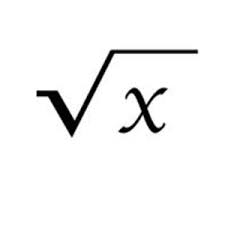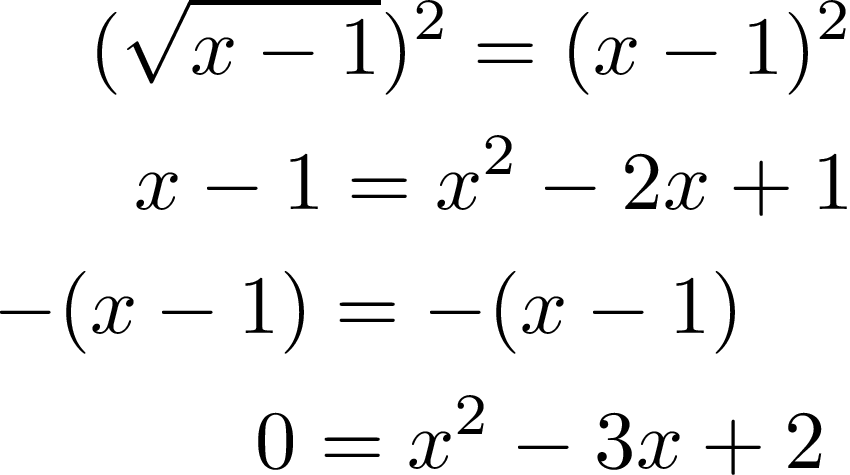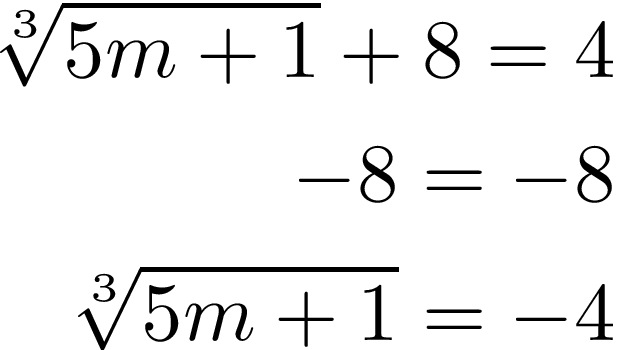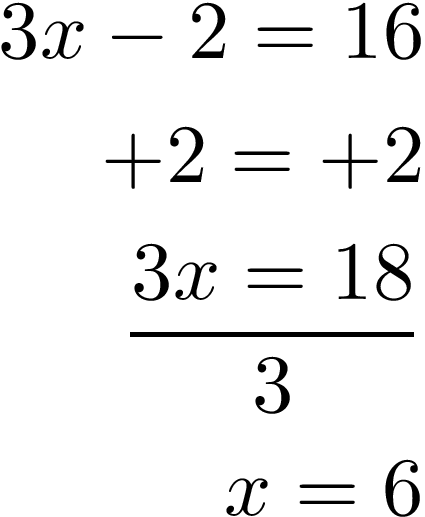This post will look at how to solve radical equations. The concepts are mostly similar to solving any other equation in terms of isolating terms etc. However, for people who are new to this, it may still be confusing. Therefore, we will go through several examples.

Example 1Our first example is a basic radical equation that includes a constant outside the radical. Below is the equation.Solving this problem requires to main steps.

2. Remove the radical by squaring it

Doing these two steps will lead to our answer. We will have two answers but the reason for this will become clear as we solve the equation.

First, we will isolate the radical by subtracting 1 from both sidesNow, to remove the radical we will square both sides. This new equation will need to be simplified and will become a quadratic equation.With our new quadratic equation we will factor this and as expected get two answers.Index Other than 2

For a radical that has an index other than  2, the process involves raising the radical to whatever power will cancel out the radical. Below is an example that has an index of 3. We will first subtract the constant from both sides.In order to remove the index of 3, we need to raise each side of the equation to the power of 3. After doing this, we solve a simple equation.One a number is a raised to a power that is a fraction it is the same as a radical. Below is an example.This means that the steps we took to solve equations with radicals can be mostly used to deal with equations with powers that are a fraction.

below is an equation. To solve this equation you must raise each side of the equation to the power of the denominator in the fraction.As you can see both sides were raised to the 4th power because that is the number in the denominator of the fraction. On the left side of the equation, the 4th power cancels out the fraction. Now you can simply solve the equation like any other equation.Hopefully, this is clear.

Conclusion

Solving radical equatons in not that diffcult. Usually, the ultimate goal is to remove the radical. The difference between this and solving for other equations is that with radical equations you want to first isolate the radical, remove the radical, and then solve for the unknown variable.